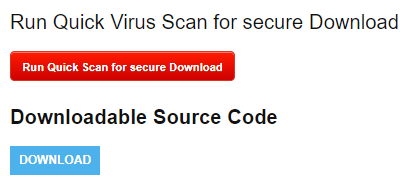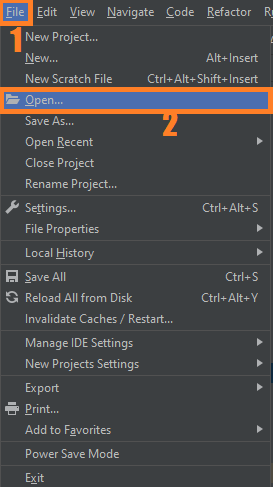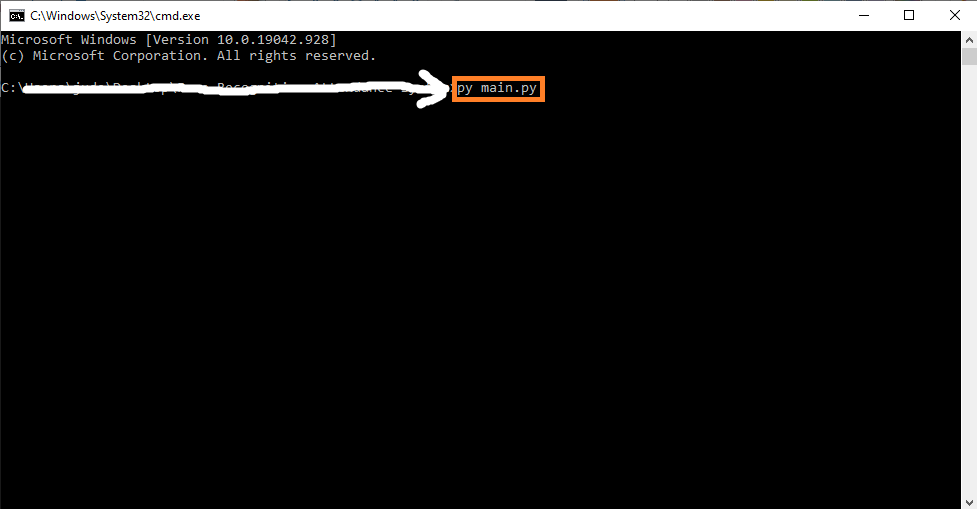# Real-Time Plate Number Detection OpenCV Python With Source Code

## Real-Time Plate Number Detection OpenCV Python With Source Code

The Real-Time Plate Number Detection OpenCV was developed using Python OpenCV, License Plate Recognition is an image-processing technology used to identify vehicles by their license plates. This technology is used in various security and traffic applications.

A Plate Number Detection OpenCV Python aims to recognize license number plates. In order to detect license number plates, we will use OpenCV to identify number plates and python pytesseract to extract characters and digits from the number plates.

By the way if you are new to python programming and you don’t know what would be the the Python IDE to use, I have here a list of Best Python IDE for Windows, Linux, Mac OS that will suit for you. I also have here How to Download and Install Latest Version of Python on Windows.

To start executing Real-Time Plate Number Detection With Source Code, make sure that you have installed Python 3.9 and PyCharm in your computer.

## Real-Time Plate Number Detection OpenCV Python With Source Code : Steps on how to run the project

Time needed: 5 minutes.

These are the steps on how to run Real-Time Plate Number Detection OpenCV Python With Source Code

First, download the given source code below and unzip the source code.• Step 2: Import the project to your PyCharm IDE.• Step 3: Run the project.

last, run the project with the command “py main.py”## Installed Libraries

```from skimage.io import imread
from skimage.filters import threshold_otsu
import matplotlib.pyplot as plt
import imutils
import cv2

from skimage import measure
from skimage.measure import regionprops
import matplotlib.pyplot as plt
import matplotlib.patches as patches
import os
import shutil```

## Complete Source Code

```from skimage.io import imread
from skimage.filters import threshold_otsu
import matplotlib.pyplot as plt
import imutils
import cv2

from skimage import measure
from skimage.measure import regionprops
import matplotlib.pyplot as plt
import matplotlib.patches as patches
import os
import shutil
filename = './plate.mp4'

if os.path.exists('output'):
shutil.rmtree('output')

os.makedirs('output')

cap = cv2.VideoCapture(filename)
# cap = cv2.VideoCapture(0)
count = 0
while cap.isOpened():
if ret == True:
cv2.imshow('window-name',frame)
cv2.imwrite("./output/frame%d.jpg" % count, frame)
count = count + 1
if cv2.waitKey(10) & 0xFF == ord('q'):
break
else:
break
cap.release()
cv2.destroyAllWindows()
#
# car image -> grayscale image -> binary image
car_image = imutils.rotate(car_image, 270)
# it should be a 2 dimensional array
print(car_image.shape)

# the next line is not compulsory however, a grey scale pixel
# in skimage ranges between 0 & 1. multiplying it with 255
# will make it range between 0 & 255 (something we can relate better with

gray_car_image = car_image * 255
fig, (ax1, ax2) = plt.subplots(1, 2)
ax1.imshow(gray_car_image, cmap="gray")
threshold_value = threshold_otsu(gray_car_image)
binary_car_image = gray_car_image > threshold_value
# print(binary_car_image)
ax2.imshow(binary_car_image, cmap="gray")
# ax2.imshow(gray_car_image, cmap="gray")
plt.show()

# CCA (finding connected regions) of binary image

# this gets all the connected regions and groups them together
label_image = measure.label(binary_car_image)

# print(label_image.shape) #width of car img

# getting the maximum width, height and minimum width and height that a license plate can be
plate_dimensions = (0.03*label_image.shape, 0.08*label_image.shape, 0.15*label_image.shape, 0.3*label_image.shape)
plate_dimensions2 = (0.08*label_image.shape, 0.2*label_image.shape, 0.15*label_image.shape, 0.4*label_image.shape)
min_height, max_height, min_width, max_width = plate_dimensions
plate_objects_cordinates = []
plate_like_objects = []

fig, (ax1) = plt.subplots(1)
ax1.imshow(gray_car_image, cmap="gray")
flag =0
# regionprops creates a list of properties of all the labelled regions
for region in regionprops(label_image):
# print(region)
if region.area < 50:
#if the region is so small then it's likely not a license plate
continue
# the bounding box coordinates
min_row, min_col, max_row, max_col = region.bbox
# print(min_row)
# print(min_col)
# print(max_row)
# print(max_col)

region_height = max_row - min_row
region_width = max_col - min_col
# print(region_height)
# print(region_width)

# ensuring that the region identified satisfies the condition of a typical license plate
if region_height >= min_height and region_height <= max_height and region_width >= min_width and region_width <= max_width and region_width > region_height:
flag = 1
plate_like_objects.append(binary_car_image[min_row:max_row,
min_col:max_col])
plate_objects_cordinates.append((min_row, min_col,
max_row, max_col))
rectBorder = patches.Rectangle((min_col, min_row), max_col - min_col, max_row - min_row, edgecolor="red",
linewidth=2, fill=False)
# let's draw a red rectangle over those regions
if(flag == 1):
# print(plate_like_objects)
plt.show()

if(flag==0):
min_height, max_height, min_width, max_width = plate_dimensions2
plate_objects_cordinates = []
plate_like_objects = []

fig, (ax1) = plt.subplots(1)
ax1.imshow(gray_car_image, cmap="gray")

# regionprops creates a list of properties of all the labelled regions
for region in regionprops(label_image):
if region.area < 50:
#if the region is so small then it's likely not a license plate
continue
# the bounding box coordinates
min_row, min_col, max_row, max_col = region.bbox
# print(min_row)
# print(min_col)
# print(max_row)
# print(max_col)

region_height = max_row - min_row
region_width = max_col - min_col
# print(region_height)
# print(region_width)

# ensuring that the region identified satisfies the condition of a typical license plate
if region_height >= min_height and region_height <= max_height and region_width >= min_width and region_width <= max_width and region_width > region_height:
# print("hello")
plate_like_objects.append(binary_car_image[min_row:max_row,
min_col:max_col])
plate_objects_cordinates.append((min_row, min_col,
max_row, max_col))
rectBorder = patches.Rectangle((min_col, min_row), max_col - min_col, max_row - min_row, edgecolor="red",
linewidth=2, fill=False)
# let's draw a red rectangle over those regions
# print(plate_like_objects)
plt.show()```